Test Description

 1 Crore+ students have signed up on EduRev. Have you?

### In the above figure, OS = OQ and PR = 2OR = 2OR, and also, OR = OS, then, PQRS is not a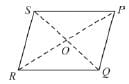∵ O is the bisector of both the diagonals.
∴ PQRS is a parallelogram.
∵ Diagonals of the parallelogram are equal.
∴ PQRS may be a square or rectangle.

### In the adjoining figure, AD || BC and AB and DC are not parallel, then ∠B =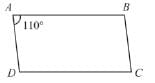∵ AB is parallel to BC, and, AB is transversal.
∴ ∠A + ∠B = 180°
⇒ ∠B = 180° - ∠A = 180° - 110° = 70°

### Find x and y: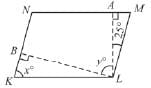KLMN is a parallelogram.

In ∆LAM,
∠ALM + 90° + ∠M = 180°
⇒∠M = 65°
∴x=∠M= 60°  (∵KLMN is a parallelogram).
In ∆BLK,
∠BLK + 65° + 90° = 180°
⇒ ∠M = 180° - 90° = 90°
⇒∠BLK = 25°
∵ ∠L = 180° - 65° = y + 25° + 25°
⇒y = 65°

ABCD is a parallelogram.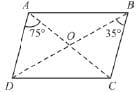The measure of ∠ADO is :

∠OBC = ∠ODA = 35°
(Alternate Interior ∠S).

The quadrilateral formed by joining the mid-points of a given quadrilateral will be (surely) :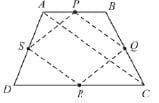ABCD is a parallelogram and P,Q,R,S are the midpoints of AB, BC, CD, DA respectively. Consider, AC as a diagonal of ABCD.
Now, According to midpoint theorem,
PQ =1/2 AC and PQ is parallel to AC,
and SR = 1/2 AC and PQ is parallel to AC.
∴ PQ = RS and PQ || RS || AC.
∴ PQRS will be a parallelogram.

ABCD is a rhombus and ABEF is a square find ‘a’.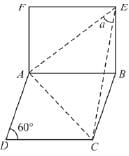∵ AB is a common side in both square and rhombus.
∴ AB = BC = CD = DA = FA = FE = EB
In ∆EBC,
∠BEC + ∠C + ∠B = 180° [∠C = ∠BEC]
[∵ EB = BC]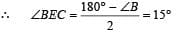[∠B = 90° + 60° = 150°]
∵ AE is the diagonal of square. ∴
∴∠AEF = 45°
Now,
∵∠ E is the angle of a square,
∴∠E = 90°
⇒ 45° + 15° + a = 90°
⇒ a = 30°

ABCD is a rectangle , with, ED = DC, ∠BOC = 120°, ∠CED = 30°.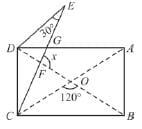Find ‘x’ from the adjoining figure.

From ∆EDC,
∠EDC = 180° - 2 × 30° = 120°
∠EDG = ∠EDC - ∠D = 120° - 90° = 30°
Also,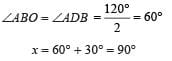The adjacent sides of a rectangle are in the ratio 5: 13 and its area is 135 cm2. The perimeter of the rectangle is :

5K × 13 K = 195
⇒ K = 3
∴  Sides of the rectangle are 15 cm, 39 cm
∴ Perimeter = 2 (15 + 39)
= 2 × 54
= 108 cm.

ABCD is a rhombus and ∠AEF = 50°. Find x.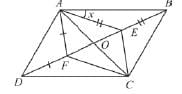∵ AE = EB.
∴ ∠BAE = ∠ABE = x.
∵ ΔDO = OB, and , AO = OC
In ΔABE,
∠DAE + ∠ABE = ∠AEF
⇒ x + x = 50°
⇒ 2x = 50°
⇒ x = 25°

ABCD is a square, P,Q,R,S  are the midpoints of AB, BC, CD and DA respectively. If the perimeter of ABCD is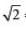cm, then perimeter of PQRS is :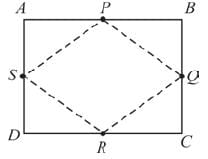In ΔAPS,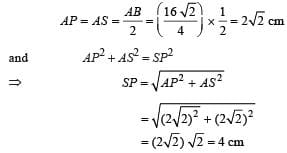∴ Perimeter (PQRS) = 4 × 4 cm
= 16 cm